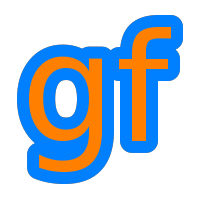Gamedev Framework (gf)  0.1.0 A C++11 framework for 2D games

Introduction

This is a quick and dirty introduction to modern OpenGL rendering pipeline. If you already know everything then you can go to the next section.

In modern OpenGL, the rendering pipeline is programmable. Not everything is programmable but the most important parts. You have to use a special language called GLSL (OpenGL Shading Language) and make programs called shaders.

The data you send to shaders is prepared in the main program and is called vertices. Vertices represent the geometry of what you have to draw. A vertex is a kind of super point: it contains the coordinates of the object but also the texture coordinates of the point, sometimes (in 3D) the normal of the surface at that point, and many more possible things.

All the vertices are sent to the graphics card that passes them to the vertex shader which is responsible of transforming the coordinates of the vertex from the game world (2D or 3D) to the screen. After this first pass, the vertices go in the rasterizer which computes the color of each pixel. Then, the pixels go through a fragment shader which can compute the final color of the pixel and sometimes other attributes.

And that's it, basically!

Rendering pipeline overview - OpenGL.org

In gf, every draw command ends in a shader. If no shader is specified, then a default shader is used.

Here is the default vertex shader:

#version 100
// The attributes of the vertex:
// - position in the game world
// - color of the vertex
// - texture coordinates
attribute vec2 a_position;
attribute vec4 a_color;
attribute vec2 a_texCoords;
// The outputs of the shader that will be passed to the fragment shader
// - color of the vertex
// - texture coordinates
varying vec4 v_color;
varying vec2 v_texCoords;
// The transformation matrix for the object.
// It is a constant (uniform) accross all vertices.
uniform mat3 u_transform;
// The main program starts here.
void main(void) {
// First, pass the texture coordinates and color to the fragment shader
v_texCoords = a_texCoords;
v_color = a_color;
// Then compute the coordinate on the screen of the vertex.
// For this, you have to compute the homogeneous coordinate of the position.
vec3 worldPosition = vec3(a_position, 1);
// And multiply by the transformation matrix.
vec3 normalizedPosition = worldPosition * u_transform;
// The output is the final position of the vertex on the screen.
gl_Position = vec4(normalizedPosition.xy, 0, 1);
}

gf::Vertex contains the data that is passed to this vertex shader. When you call gf::RenderTarget::draw(), you have to provide an array of gf::Vertex which is then handled by the shader:

• The position attribute becomes a_position in the shader
• The color attribute becomes a_color in the shader
• The texCoords attribute becomes a_texCoords in the shader

The u_transform constant is set by the library automatically. It is computed from the global transform matrix put in gf::RenderStates and from the object transform matrix (see gf::Transformable::getTransform()).

Here is the default fragment shader:

#version 100
precision mediump float;
// The inputs from the vertex shader
// - color of the vertex
// - texture coordinates
varying vec4 v_color;
varying vec2 v_texCoords;
// The texture used for the object
// It is a constant (uniform) accross all vertices.
uniform sampler2D u_texture;
// The main program starts here.
void main(void) {
// Compute the color from the texture and texture coordinates
vec4 color = texture2D(u_texture, v_texCoords);
// Compute the final color by multiplying with the color of the object
gl_FragColor = color * v_color;
}

The u_texture constant is set by the library automatically. If no texture is provided, a default opaque white infinite texture is set.

Quite easy. We are in 2D!

If you want to write your own shader, you will have to use the same variables (with the same names) so that you can receive the data from the vertices. The easiest way to do it is to start from the default shaders and modify them. This means you can not add attribute variables, but you can do whatever you want with varying variables and you can add uniform variables as long as you keep the two already present variables u_transform and u_texture.

If you have additional constants (uniforms), you can set them with one of the gf::Shader::setUniform() functions.

gf::Sprite sprite;
// ... initialize the sprite
renderer.draw(sprite, states);

Post-processing

Post-processing is an application of global graphic effects after the frame has been computed. It can be used for a wide range of usage. Technically, the frame is rendered in a texture and the texture is then sent into the graphics pipeline again i.e. in the shaders where you can apply the desired effect.